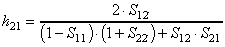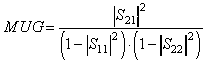Next: 3.2 Small Signal Equivalent Circuit Parameter Extraction Up: 3 Measurement and Parameter Extraction Previous: 3 Measurement and Parameter Extraction

3.1 DC and RF Measurements

The DC and RF characteristics of fabricated HEMTs are determined by measuring the voltages and currents on the three terminals and 2­port S­parameters. One of the most important figures of merit are the current gain cut-off frequency fT and the maximum oscillation frequency fmax. fT is defined as the frequency f were the current gain |h21| gets 0 dB. The current gain can be calculated from the measured S­parameters by :. (8)

If |h21| [dB] is positive in the whole measured frequency range |h21| [dB]is linearly extrapolated to zero versus log(f) with a slope of 20dB/dec. fmax is defined as the frequency at which the unilateral gain MUG gets zero. MUG can be obtained from S­parameters by :. (9)

Helmut Brech
1998-03-11